# Error correcting codes

## Parity Check code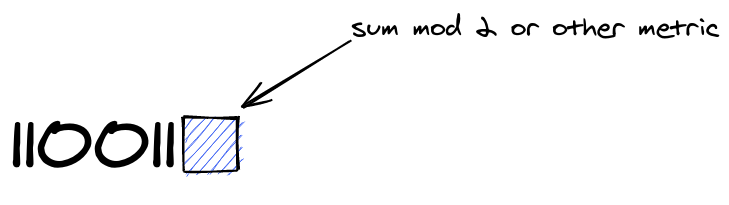Can only detect one error and cannot correct any errors

## Repetition Code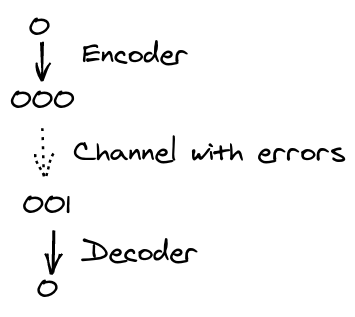Can correct

\$\$\bigg\lfloor \dfrac{n-1}{2} \bigg\rfloor\$\$

errors

and can detect \$n-1\$ errors

## Hamming distance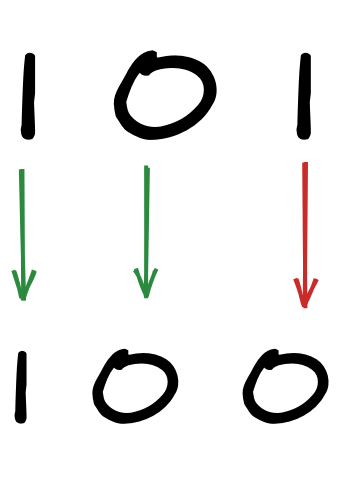Measures the number of differences (in this case 1)

The Hamming weight is the number of non zeroes (2 for top, 1 for bottom)

## Minimum distance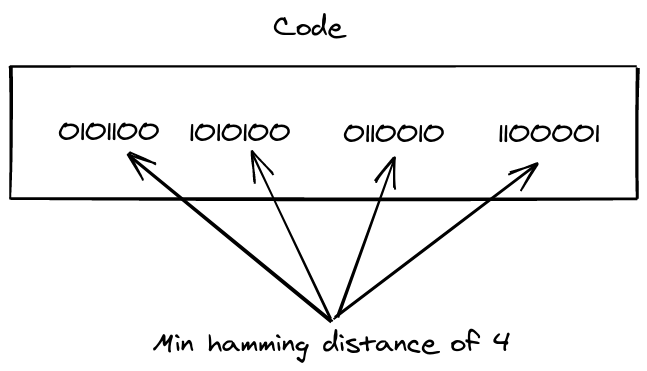A code can correct t errors iff it has a minimum distance

\$\$d_{min}\geqslant 2t+1\$\$

So for this code it can correct 1 error (min distance of 3 would also work to correct 1 error)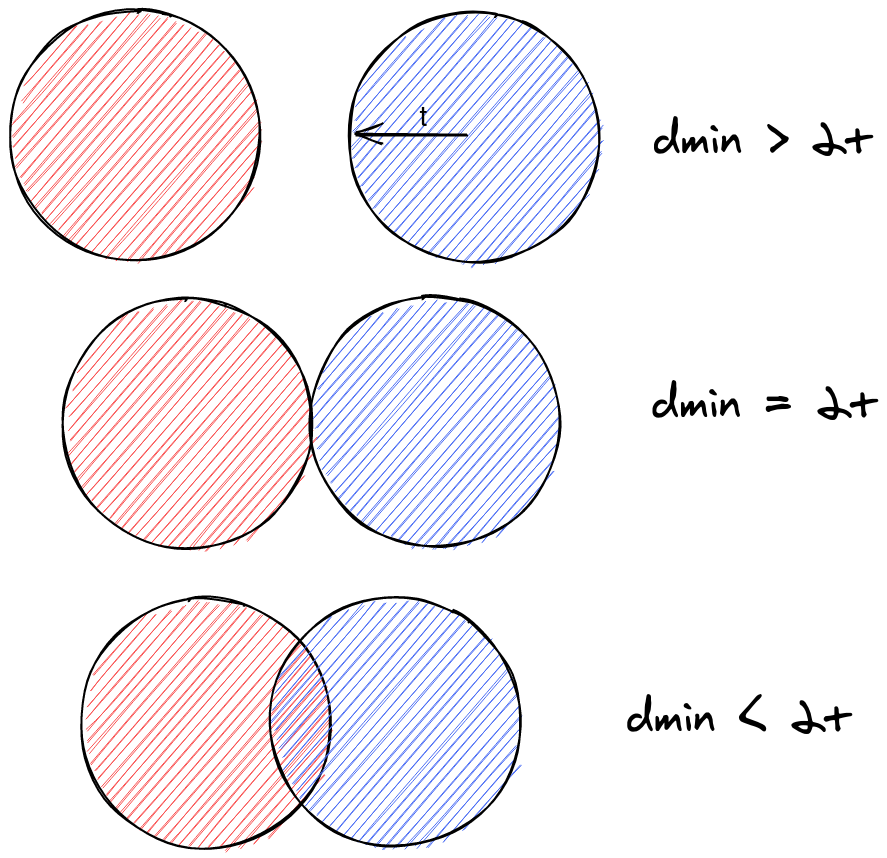There needs to be overlap between the codes in order to be able to distinguish them

As the \$d_{min}\$ is an integer value, the formula is equivalent to \$d_{min}>2t\$